Refer to our Texas Go Math Grade 1 Answer Key Pdf to score good marks in the exams. Test yourself by practicing the problems from Texas Go Math Grade 1 Lesson 13.3 Answer Key Use Addition to Check Subtraction.

Explore

Draw and write to solve the problem.FOR THE TEACHER • Read the problem. Erin has 11 books. I borrow 4 of them. How many books does Erin still have? Allow children time to solve, using the top workspace. Then read this part of the problem: I give 4 books back to Erin. How many books does Erin have now?
Given that
Total number of books at Erin = 11 books
I borrow books = 4
Total number of books near Erin still = 11 – 4 = 7
I gave 4 books back to Erin = 7 + 4 = 11.

Math Talk
Mathematical Processes

Does Erin get all her books back? Use the number sentences to explain how you know.

Model and Draw

Why can you use addition to check subtraction?Share and Check

Question 1.Question 2.Question 3.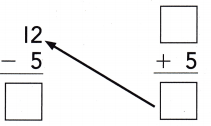Question 4.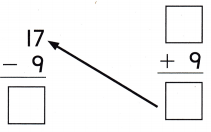Problem Solving

Question 5.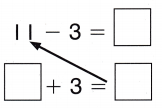Question 6.Question 7.Question 8.Question 9.
H.O.T. Brianna has 13 sand dollars. Some sand dollars are broken. 5 sand dollars are not broken. Write number sentences about the sand dollars.Given that
Brianna has 13 sand dollars.
Some of the sand dollars are broken = 5
Some of the sand dollars are not broken = 13 – 5 = 8

Question 10.
Liam brings 15 cupcakes to the party. His friends eat all but 6 of them. How many cupcakes did they eat?____________ cupcakes
Given that
Liam brings cupcakes to the party = 15
Some of these friends are eaten and the remaining are 6
Cupcakes eaten by friends = 15 – 6 = 9.

Question 11.
Analyze 12 rabbits are in the pen. 3 rabbits are given away. 9 rabbits are left. Which addition sentence can you use to check the subtraction?(A) 8 + 3 = 11
(B) 3 + 9 = 12
(C) 12 + 3 = 15
Given that
Rabbits are in the pen = 12
Rabbits are given away = 3
Rabbits are left = 9
3 + 9 = 12 addition sentence is used to check the subtraction.
Option B is correct.

Question 12.
There are 13 ladybugs on a plant. Then 7 fly away. 6 ladybugs are left. Which fact can you use to check the subtraction?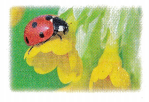(A) 6 + 7 = 13
(B) 13 – 8 = 5
(C) 7 – 6 = 1
Given that
Ladybugs on a plant = 13
6 + 7 = 13 fact is used to check the subtraction

Question 13.
Multi-Step There are 15 people on a bus. 8 people get off. How many people are left? Which addition sentence can you use to check the subtraction?
(A) 15 + 8 = 23
(B) 8 + 9 = 17
(C) 7 + 8 = 15

Total number of people on a bus = 15
Total number of people get off = 8
People left in bus = 15 – 8 = 7
7 + 8 = 15 is related to check the subtraction
Option C is the correct answer.

Question 14.
Texas Test Prep Which addition sentence can you use to check the subtraction?
11 – 2 = __________
(A) 9 + 2 = 11
(B) 7 + 8 = 15
(C) 6 + 2 = 8
11 – 2 = 9
9 + 2 = 11 addition sentence is used to check the subtraction.

TAKE HOME ACTIVITY • Write 11 – 7 = _________ on a sheet of paper. Ask your child to find the difference and then write an addition sentence he or she can use to check the subtraction.
11 – 7 = 4
Difference = 4
Addition sentence = 7 + 4 = 11

### Texas Go Math Grade 1 Lesson 13.3 Homework and Practice Answer Key

Question 1.Question 2.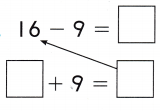Question 3.Question 4.Problem Solving

Question 5.
Marty has 14 stickers. Some stickers are torn. 8 stickers are not torn. Write number sentences about the stickers.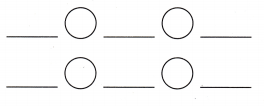Given that
Marty has stickers = 14
Stickers are not torn = 8
Stickers are torn = 14 – 8 = 6

Lesson Check

Question 6.
14 flowers are on a bush. Mike picks 6 flowers. 8 flowers are left on the bush. Which addition sentence can you use to check the subtraction?(A) 6 + 8 = 14
(B) 7 + 6 = 13
(C) 14 + 4 = 18
Given that
Flowers are on the bush = 14
Mike picks flowers = 6
Flowers left on the bush = 8
6 + 8 = 14 addition subtraction is used.

Question 7.
There are 17 children on a trip to the zoo. q children go to see the monkeys. The rest go to see the snakes. Which fact can you use to check the subtraction?
17 – 9 = ___________17 – 9 = 8

Question 8.
Which addition sentence can you use to check the subtraction?
12 – 5 = __________
(A) 6 + 6 = 12
(B) 7 + 5 = 12
(C) 9 + 5 = 14
12 – 5 = 7
7 + 5 = 12 addition sentence can be used to check the subtraction.

Question 9.
Multi-step There are 15 children on the playground. 6 children go inside. How many children are left? Which addition sentence can you use to check the subtraction?
(A) 15 + 6 = 2
(B) 6 + 9 = 15
(C) 6 + 8 = 14